## [Paper published] Check out our new (deep) machine learning paper for flood detection

New machine/deep learning paper led by Liping: Analysis of remote sensing imagery for disaster assessment using deep learning: a case study of flooding event

A full-text view-only version of the paper can be found via the link: https://rdcu.be/bpUvx.## Machine Learning Algorithms Mini-Course

source: from machinelearningmastery.com  (Good posts sometimes disappear, so I repost it here for my and for your information.)

Machine learning algorithms are a very large part of machine learning.

You have to understand how they work to make any progress in the field.

In this post you will discover a 14-part machine learning algorithms mini course that you can follow to finally understand machine learning algorithms.

We are going to cover a lot of ground in this course and you are going to have a great time. Let’s get started.Machine Learning Algorithms Mini-Course
Photo by Jared Tarbell, some rights reserved.

## Who is This Course For?

Before we get started, let’s make sure you are in the right place.

• This course is for beginners curious about machine learning algorithms.
• This course does not assume you know how to write code.
• This course does not assume a background in mathematics.
• This course does not assume a background in machine learning theory.

This mini-course will take you on a guided tour of machine learning algorithms from foundations  and through 10 top techniques.

We will visit each algorithm to give you a sense of how it works, but not go into too much depth to keep things moving.

## Mini-Course Overview

Let’s take a look at what we’re going to cover over the next 14 lessons.

You may need to come back to this post again and again, so you may want to bookmark it.

This mini-course is broken down int four parts: Algorithm Foundations, Linear Algorithms, Nonlinear Algorithms and Ensemble Algorithms.

### Algorithm Foundations

• Lesson 1: How To Talk About Data in Machine Learning
• Lesson 2: Principle That Underpins All Algorithms
• Lesson 3: Parametric and Nonparametric Algorithms
• Lesson 4: Bias, Variance and the Trade-off

### Linear Algorithms

• Lesson 5: Linear Regression
• Lesson 6: Logistic Regression
• Lesson 7: Linear Discriminant Analysis

### Nonlinear Algorithms

• Lesson 8: Classification and Regression Trees
• Lesson 9: Naive Bayes
• Lesson 10: k-Nearest Neighbors
• Lesson 11: Learning Vector Quantization
• Lesson 12: Support Vector Machines

### Ensemble Algorithms

• Lesson 13: Bagging and Random Forest
• Lesson 14: Boosting and AdaBoost

## Lesson 1: How To Talk About Data in Machine Learning

Data plays a big part in machine learning.

It is important to understand and use the right terminology when talking about data.

How do you think about data? Think of a spreadsheet. You have columns, rows, and cells.

The statistical perspective of machine learning frames data in the context of a hypothetical function (f) that the machine learning algorithm aims to learn. Given some input variables (Input)  the function answer the question as to what is the predicted output variable (Output).

Output = f(Input)

The inputs and outputs can be referred to as variables or vectors.

The computer science perspective uses a row of data to describe an entity (like a person) or an observation about an entity. As such, the columns for a row are often referred to as attributes of the observation and the rows themselves are called instances.

## Lesson 2: The Principle That Underpins All Algorithms

There is a common principle that underlies all supervised machine learning algorithms for predictive modeling.

Machine learning algorithms are described as learning a target function (f) that best maps input variables (X) to an output variable (Y).

Y = f(X)

This is a general learning task where we would like to make predictions in the future (Y) given new examples of input variables (X). We don’t know what the function (f) looks like or it’s form. If we did, we would use it directly and we would not need to learn it from data using machine learning algorithms.

The most common type of machine learning is to learn the mapping Y = f(X) to make predictions of Y for new X. This is called predictive modeling or predictive analytics and our goal is to make the most accurate predictions possible.

## Lesson 3: Parametric and Nonparametric Algorithms

What is a parametric machine learning algorithm and how is it different from a nonparametric machine learning algorithm?

Assumptions can greatly simplify the learning process, but can also limit what can be learned. Algorithms that simplify the function to a known form are called parametric machine learning algorithms.

The algorithms involve two steps:

1. Select a form for the function.
2. Learn the coefficients for the function from the training data.

Some examples of parametric machine learning algorithms are Linear Regression and Logistic Regression.

Algorithms that do not make strong assumptions about the form of the mapping function are called nonparametric machine learning algorithms. By not making assumptions, they are free to learn any functional form from the training data.

Non-parametric methods are often more flexible, achieve better accuracy but require a lot more data and training time.

Examples of nonparametric algorithms include Support Vector Machines, Neural Networks and Decision Trees.

## Lesson 4: Bias, Variance and the Trade-off

Machine learning algorithms can best be understood through the lens of the bias-variance trade-off.

Bias are the simplifying assumptions made by a model to make the target function easier to learn.

Generally parametric algorithms have a high bias making them fast to learn and easier to understand but generally less flexible. In turn they have lower predictive performance on complex problems that fail to meet the simplifying assumptions of the algorithms bias.

Decision trees are an example of a low bias algorithm, whereas linear regression is an example of a high-bias algorithm.

Variance is the amount that the estimate of the target function will change if different training data was used. The target function is estimated from the training data by a machine learning algorithm, so we should expect the algorithm to have some variance, not zero variance.

The k-Nearest Neighbors algorithm is an example of a high-variance algorithm, whereas Linear Discriminant Analysis is an example of a low variance algorithm.

The goal of any predictive modeling machine learning algorithm is to achieve low bias and low variance. In turn the algorithm should achieve good prediction performance. The parameterization of machine learning algorithms is often a battle to balance out bias and variance.

• Increasing the bias will decrease the variance.
• Increasing the variance will decrease the bias.

## Lesson 5: Linear Regression Algorithm

Linear regression is perhaps one of the most well known and well understood algorithms in statistics and machine learning.

Isn’t it a technique from statistics?

Predictive modeling is primarily concerned with minimizing the error of a model or making the most accurate predictions possible, at the expense of explainability. We will borrow, reuse and steal algorithms from many different fields, including statistics and use them towards these ends.

The representation of linear regression is a equation that describes a line that best fits the relationship between the input variables (x) and the output variables (y), by finding specific weightings for the input variables called coefficients (B).

For example:

y = B0 + B1 * x

We will predict y given the input x and the goal of the linear regression learning algorithm is to find the values for the coefficients B0 and B1.

Different techniques can be used to learn the linear regression model from data, such as a linear algebra solution for ordinary least squares and gradient descent optimization.

Linear regression has been around for more than 200 years and has been extensively studied. Some good rules of thumb when using this technique are to remove variables that are very similar (correlated) and to remove noise from your data, if possible.

It is a fast and simple technique and good first algorithm to try.

## Lesson 6: Logistic Regression Algorithm

Logistic regression is another technique borrowed by machine learning from the field of statistics. It is the go-to method for binary classification problems (problems with two class values).

Logistic regression is like linear regression in that the goal is to find the values for the coefficients that weight each input variable.

Unlike linear regression, the prediction for the output is transformed using a non-linear function called the logistic function.

The logistic function looks like a big S and will transform any value into the range 0 to 1. This is useful because we can apply a rule to the output of the logistic function to snap values to 0 and 1 (e.g. IF less than 0.5 then output 1) and predict a class value.

Because of the way that the model is learned, the predictions made by logistic regression can also be used as the probability of a given data instance belonging to class 0 or class 1. This can be useful on problems where you need to give more rationale for a prediction.

Like linear regression, logistic regression does work better when you remove attributes that are unrelated to the output variable as well as attributes that are very similar (correlated) to each other.

It’s a fast model to learn and effective on binary classification problems.

## Lesson 7: Linear Discriminant Analysis Algorithm

Logistic regression is a classification algorithm traditionally limited to only two-class classification problems. If you have more than two classes then the Linear Discriminant Analysis algorithm is the preferred linear classification technique.

The representation of LDA is pretty straight forward. It consists of statistical properties of your data, calculated for each class. For a single input variable this includes:

1. The mean value for each class.
2. The variance calculated across all classes.

Predictions are made by calculating a discriminate value for each class and making a prediction for the class with the largest value.

The technique assumes that the data has a Gaussian distribution (bell curve), so it is a good idea to remove outliers from your data before hand.

It’s a simple and powerful method for classification predictive modeling problems.

## Lesson 8: Classification and Regression Trees

Decision Trees are an important type of algorithm for predictive modeling machine learning.

The representation for the decision tree model is a binary tree. This is your binary tree from algorithms and data structures, nothing too fancy. Each node represents a single input variable (x) and a split point on that variable (assuming the variable is numeric).

The leaf nodes of the tree contain an output variable (y) which is used to make a prediction.  Predictions are made by walking the splits of the tree until arriving at a leaf node and output the class value at that leaf node.

Trees are fast to learn and very fast for making predictions. They are also often accurate for a broad range of problems and do not require any special preparation for your data.

Decision trees have a high variance and can yield more accurate predictions when used in an ensemble, a topic we will cover in Lesson 13 and Lesson 14.

## Lesson 9: Naive Bayes Algorithm

Naive Bayes is a simple but surprisingly powerful algorithm for predictive modeling.

The model is comprised of two types of probabilities that can be calculated directly from your training data:

1. The probability of each class.
2. The conditional probability for each class given each x value.

Once calculated, the probability model can be used to make predictions for new data using Bayes Theorem.

When your data is real-valued it is common to assume a Gaussian distribution (bell curve) so that you can easily estimate these probabilities.

Naive Bayes is called naive because it assumes that each input variable is independent. This is a strong assumption and unrealistic for real data, nevertheless, the technique is very effective on a large range of complex problems.

## Lesson 10: K-Nearest Neighbors Algorithm

The KNN algorithm is very simple and very effective.

The model representation for KNN is the entire training dataset. Simple right?

Predictions are made for a new data point by searching through the entire training set for the K most similar instances (the neighbors) and summarizing the output variable for those K instances. For regression this might be the mean output variable, in classification this might be the mode (or most common) class value.

The trick is in how to determine similarity between the data instances. The simplest technique if your attributes are all of the same scale (all in inches for example) is to use the Euclidean distance, a number you can calculate directly based on the differences between each input variable.

KNN can require a lot of memory or space to store all of the data, but only performs a calculation (or learn) when a prediction is needed, just in time. You can also update and curate your training instances over time to keep predictions accurate.

The idea of distance or closeness can break down in very high dimensions (lots of input variables) which can negatively effect the performance of the algorithm on your problem. This is called the curse of dimensionality. It suggests you only use those input variables that are most relevant to predicting the output variable.

## Lesson 11: Learning Vector Quantization

A downside of K-Nearest Neighbors is that you need to hang on to your entire training dataset.

The Learning Vector Quantization algorithm (or LVQ for short) is an artificial neural network algorithm that allows you to choose how many training instances to hang onto and learns exactly what those instances should look like.

The representation for LVQ is a collection of codebook vectors. These are selected randomly in the beginning and adapted to best summarize the training dataset over a number of iterations of the learning algorithm.

After learned, the codebook vectors can be used to make predictions just like K-Nearest Neighbors. The most similar neighbor (best matching codebook vector) is found by calculating the distance between each codebook vector and the new data instance. The class value or (real value in the case of regression) for the best matching unit is then returned as the prediction.

Best results are achieved if you rescale your data to have the same range, such as between 0 and 1.

If you discover that KNN gives good results on your dataset try using LVQ to reduce the memory requirements of storing the entire training dataset.

## Lesson 12: Support Vector Machines

Support Vector Machines are perhaps one of the most popular and talked about machine learning algorithms.

A hyperplane is a line that splits the input variable space. In SVM, a hyperplane is selected to best separate the points in the input variable space by their class, either class 0 or class 1.

In two-dimensions you can visualize this as a line and let’s assume that all of our input points can be completely separated by this line.

The SVM learning algorithm finds the coefficients that results in the best separation of the classes by the hyperplane.

The distance between the hyperplane and the closest data points is referred to as the margin. The best or optimal hyperplane that can separate the two classes is the line that as the largest margin.

Only these points are relevant in defining the hyperplane and in the construction of the classifier.

These points are called the support vectors. They support or define the hyperplane.

In practice, an optimization algorithm is used to find the values for the coefficients that maximizes the margin.

SVM might be one of the most powerful out-of-the-box classifiers and worth trying on your dataset.

## Lesson 13: Bagging and Random Forest

Random Forest is one of the most popular and most powerful machine learning algorithms. It is a type of ensemble machine learning algorithm called Bootstrap Aggregation or bagging.

The bootstrap is a powerful statistical method for estimating a quantity from a data sample. Such as a mean. You take lots of samples of your data, calculate the mean, then average all of your mean values to give you a better estimation of the true mean value.

In bagging, the same approach is used, but instead for estimating entire statistical models, most commonly decision trees.

Multiple samples of your training data are taken then models are constructed for each data sample. When you need to make a prediction for new data, each model makes a prediction and the predictions are averaged to give a better estimate of the true output value.

Random forest is a tweak on this approach where decision trees are created so that rather than selecting optimal split points, suboptimal splits are made by introducing randomness.

The models created for each sample of the data are therefore more different than they otherwise would be, but still accurate in their unique and different ways. Combining their predictions results in a better estimate of the true underlying output value.

If you get good good results with an algorithm with high variance (like decision trees), you can often get better results by bagging that algorithm.

## Lesson 14: Boosting and AdaBoost

Boosting is an ensemble technique that attempts to create a strong classifier from a number of weak classifiers.

This is done by building a model from the training data, then creating a second model that attempts to correct the errors from the first model. Models are added until the training set is predicted perfectly or a maximum number of models are added.

AdaBoost was the first really successful boosting algorithm developed for binary classification. It is the best starting point for understanding boosting. Modern boosting methods build on AdaBoost, most notably stochastic gradient boosting machines.

AdaBoost is used with short decision trees. After the first tree is created, the performance of the tree on each training instance is used to weight how much attention the next tree that is created should pay attention to each training instance. Training data that is hard to predict is given more more weight, whereas easy to predict instances are given less weight.

Models are created sequentially one after the other, each updating the weights on the training instances that affect the learning performed by the next tree in the sequence.

After all the trees are built, predictions are made for new data, and the performance of each tree is weighted by how accurate it was on the training data.

Because so much attention is put on correcting mistakes by the algorithm it is important that you have clean data with outliers removed.

## Mini-Course Review

You made it. Well done! Take a moment and look back at how far you have come:

• You discovered how to talk about data in machine learning and about the underlying principles of all predictive modeling algorithms.
• You discovered the difference between parametric and nonparametric algorithms and the difference between error introduced by bias and variance.
• You discovered three linear machine learning algorithms: Linear Regression, Logistic Regression and Linear Discriminant Analysis.
• You were introduced to 5 nonlinear algorithms: Classification and Regression Trees, Naive Bayes, K-Nearest Neighbors, Learning Vector Quantization and Support Vector Machines.
• Finally, you discovered two of the most popular ensemble algorithms: Bagging with Decision Trees and Boosting with AdaBoost.

Don’t make light of this, you have come a long way in a short amount of time. This is just the beginning of your journey with machine learning algorithms. Keep practicing and developing your skills.

## The 10 Algorithms Machine Learning Engineers Need to Know

Source: from kdnuggets.com (Good posts sometimes disappear, so I repost it here for my and for your information.)

Read this introductory list of contemporary machine learning algorithms of importance that every engineer should understand.

By James Le, New Story Charity.It is no doubt that the sub-field of machine learning / artificial intelligence has increasingly gained more popularity in the past couple of years. As Big Data is the hottest trend in the tech industry at the moment, machine learning is incredibly powerful to make predictions or calculated suggestions based on large amounts of data. Some of the most common examples of machine learning are Netflix’s algorithms to make movie suggestions based on movies you have watched in the past or Amazon’s algorithms that recommend books based on books you have bought before.

So if you want to learn more about machine learning, how do you start? For me, my first introduction is when I took an Artificial Intelligence class when I was studying abroad in Copenhagen. My lecturer is a full-time Applied Math and CS professor at the Technical University of Denmark, in which his research areas are logic and artificial, focusing primarily on the use of logic to model human-like planning, reasoning and problem solving. The class was a mix of discussion of theory/core concepts and hands-on problem solving. The textbook that we used is one of the AI classics: Peter Norvig’s Artificial Intelligence — A Modern Approach, in which we covered major topics including intelligent agents, problem-solving by searching, adversarial search, probability theory, multi-agent systems, social AI, philosophy/ethics/future of AI. At the end of the class, in a team of 3, we implemented simple search-based agents solving transportation tasks in a virtual environment as a programming project.

I have learned a tremendous amount of knowledge thanks to that class, and decided to keep learning about this specialized topic. In the last few weeks, I have been multiple tech talks in San Francisco on deep learning, neural networks, data architecture — and a Machine Learning conference with a lot of well-known professionals in the field. Most importantly, I enrolled inUdacity’s Intro to Machine Learning online course in the beginning of June and has just finished it a few days ago. In this post, I want to share some of the most common machine learning algorithms that I learned from the course.

Machine learning algorithms can be divided into 3 broad categories — supervised learning, unsupervised learning, and reinforcement learning.Supervised learning is useful in cases where a property (label) is available for a certain dataset (training set), but is missing and needs to be predicted for other instances. Unsupervised learning is useful in cases where the challenge is to discover implicit relationships in a given unlabeled dataset (items are not pre-assigned). Reinforcement learning falls between these 2 extremes — there is some form of feedback available for each predictive step or action, but no precise label or error message. Since this is an intro class, I didn’t learn about reinforcement learning, but I hope that 10 algorithms on supervised and unsupervised learning will be enough to keep you interested.

### Supervised Learning

1. Decision Trees: A decision tree is a decision support tool that uses a tree-like graph or model of decisions and their possible consequences, including chance-event outcomes, resource costs, and utility. Take a look at the image to get a sense of how it looks like.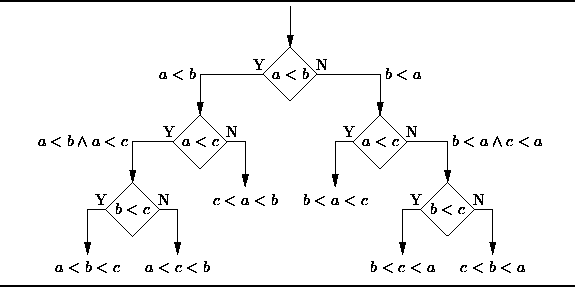Decision Tree
From a business decision point of view, a decision tree is the minimum number of yes/no questions that one has to ask, to assess the probability of making a correct decision, most of the time. As a method, it allows you to approach the problem in a structured and systematic way to arrive at a logical conclusion.

2. Naïve Bayes Classification: Naïve Bayes classifiers are a family of simple probabilistic classifiers based on applying Bayes’ theorem with strong (naïve) independence assumptions between the features. The featured image is the equation — with P(A|B) is posterior probability, P(B|A) is likelihood, P(A) is class prior probability, and P(B) is predictor prior probability.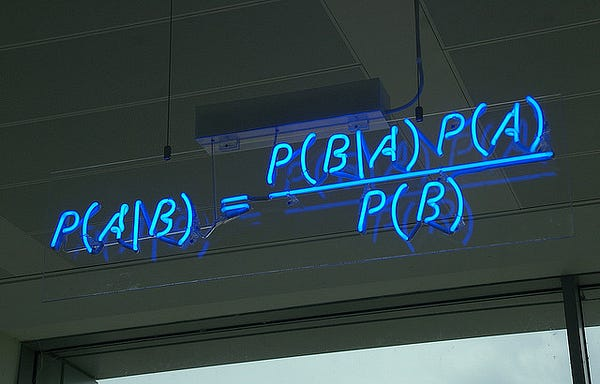Naive Bayes Classification
Some of real world examples are:

• To mark an email as spam or not spam
• Classify a news article about technology, politics, or sports
• Check a piece of text expressing positive emotions, or negative emotions?
• Used for face recognition software.

3. Ordinary Least Squares Regression: If you know statistics, you probably have heard of linear regression before. Least squares is a method for performing linear regression. You can think of linear regression as the task of fitting a straight line through a set of points. There are multiple possible strategies to do this, and “ordinary least squares” strategy go like this — You can draw a line, and then for each of the data points, measure the vertical distance between the point and the line, and add these up; the fitted line would be the one where this sum of distances is as small as possible.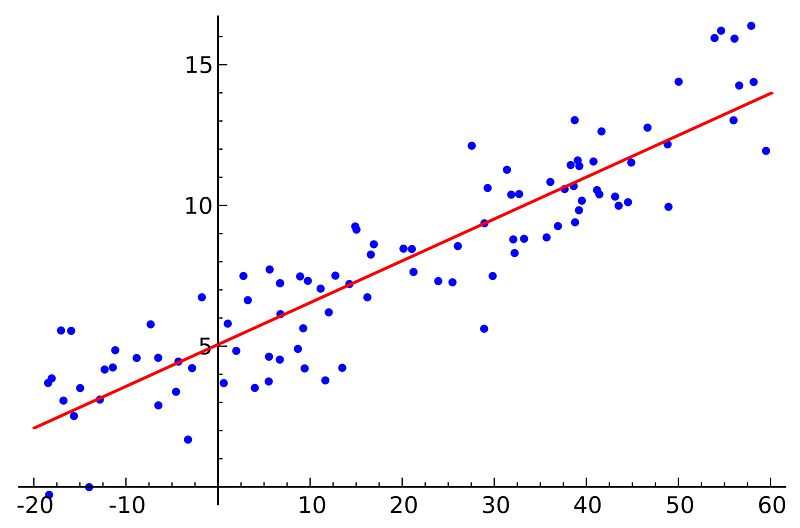Ordinary Least Squares Regression
Linear refers the kind of model you are using to fit the data, while least squares refers to the kind of error metric you are minimizing over.

4. Logistic Regression: Logistic regression is a powerful statistical way of modeling a binomial outcome with one or more explanatory variables. It measures the relationship between the categorical dependent variable and one or more independent variables by estimating probabilities using a logistic function, which is the cumulative logistic distribution.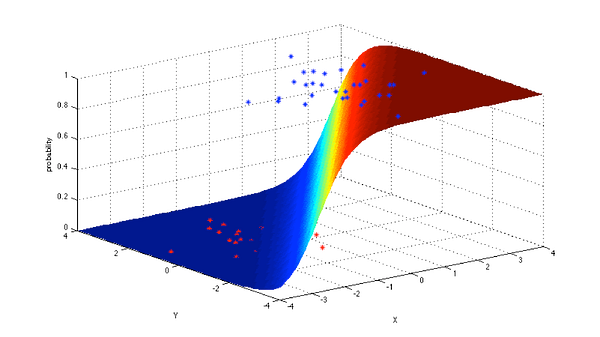Logistic Regression
In general, regressions can be used in real-world applications such as:

• Credit Scoring
• Measuring the success rates of marketing campaigns
• Predicting the revenues of a certain product
• Is there going to be an earthquake on a particular day?

5. Support Vector Machines: SVM is binary classification algorithm. Given a set of points of 2 types in N dimensional place, SVM generates a (N — 1) dimensional hyperlane to separate those points into 2 groups. Say you have some points of 2 types in a paper which are linearly separable. SVM will find a straight line which separates those points into 2 types and situated as far as possible from all those points.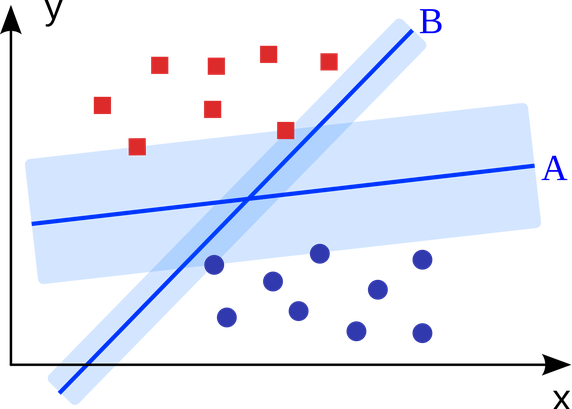Support Vector Machine
In terms of scale, some of the biggest problems that have been solved using SVMs (with suitably modified implementations) are display advertising, human splice site recognition, image-based gender detection, large-scale image classification…

6. Ensemble Methods: Ensemble methods are learning algorithms that construct a set of classifiers and then classify new data points by taking a weighted vote of their predictions. The original ensemble method is Bayesian averaging, but more recent algorithms include error-correcting output coding, bagging, and boosting.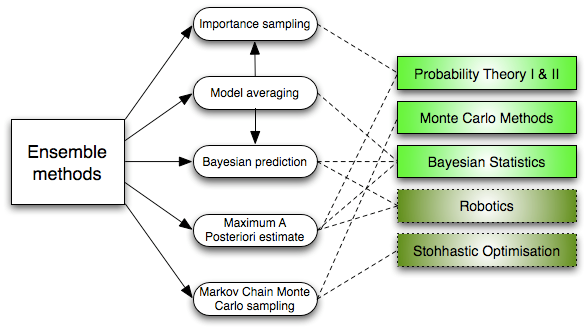Ensemble Learning Algorithms
So how do ensemble methods work and why are they superior to individual models?

• They average out biases: If you average a bunch of democratic-leaning polls and republican-leaning polls together, you will get an average something that isn’t leaning either way.
• They reduce the variance: The aggregate opinion of a bunch of models is less noisy than the single opinion of one of the models. In finance, this is called diversification — a mixed portfolio of many stocks will be much less variable than just one of the stocks alone. This is why your models will be better with more data points rather than fewer.
• They are unlikely to over-fit: If you have individual models that didn’t over-fit, and you are combining the predictions from each model in a simple way (average, weighted average, logistic regression), then there’s no room for over-fitting.

### Unsupervised Learning

7. Clustering Algorithms: Clustering is the task of grouping a set of objects such that objects in the same group (cluster) are more similar to each other than to those in other groups.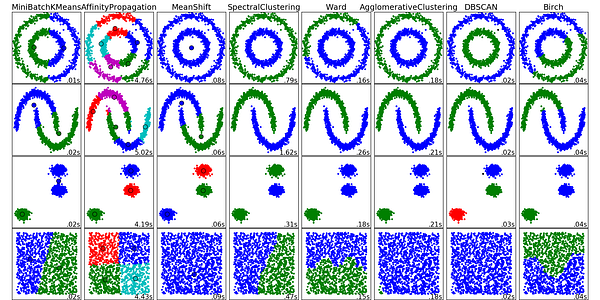Clustering Algorithms
Every clustering algorithm is different, and here are a couple of them:

• Centroid-based algorithms
• Connectivity-based algorithms
• Density-based algorithms
• Probabilistic
• Dimensionality Reduction
• Neural networks / Deep Learning

8. Principal Component Analysis: PCA is a statistical procedure that uses an orthogonal transformation to convert a set of observations of possibly correlated variables into a set of values of linearly uncorrelated variables called principal components.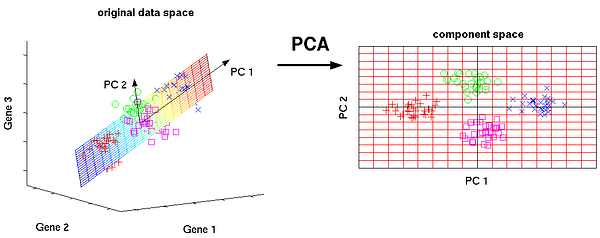Principal Component Analysis
Some of the applications of PCA include compression, simplifying data for easier learning, visualization. Notice that domain knowledge is very important while choosing whether to go forward with PCA or not. It is not suitable in cases where data is noisy (all the components of PCA have quite a high variance).

9. Singular Value Decomposition: In linear algebra, SVD is a factorization of a real complex matrix. For a given m * n matrix M, there exists a decomposition such that M = UΣV, where U and V are unitary matrices and Σ is a diagonal matrix.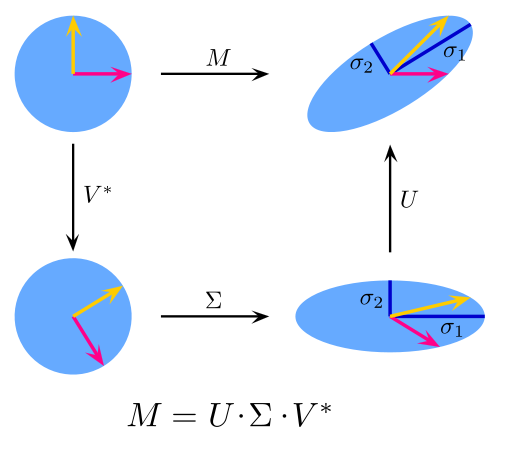Singular Value Decomposition
PCA is actually a simple application of SVD. In computer vision, the 1st face recognition algorithms used PCA and SVD in order to represent faces as a linear combination of “eigenfaces”, do dimensionality reduction, and then match faces to identities via simple methods; although modern methods are much more sophisticated, many still depend on similar techniques.

10. Independent Component Analysis: ICA is a statistical technique for revealing hidden factors that underlie sets of random variables, measurements, or signals. ICA defines a generative model for the observed multivariate data, which is typically given as a large database of samples. In the model, the data variables are assumed to be linear mixtures of some unknown latent variables, and the mixing system is also unknown. The latent variables are assumed non-gaussian and mutually independent, and they are called independent components of the observed data.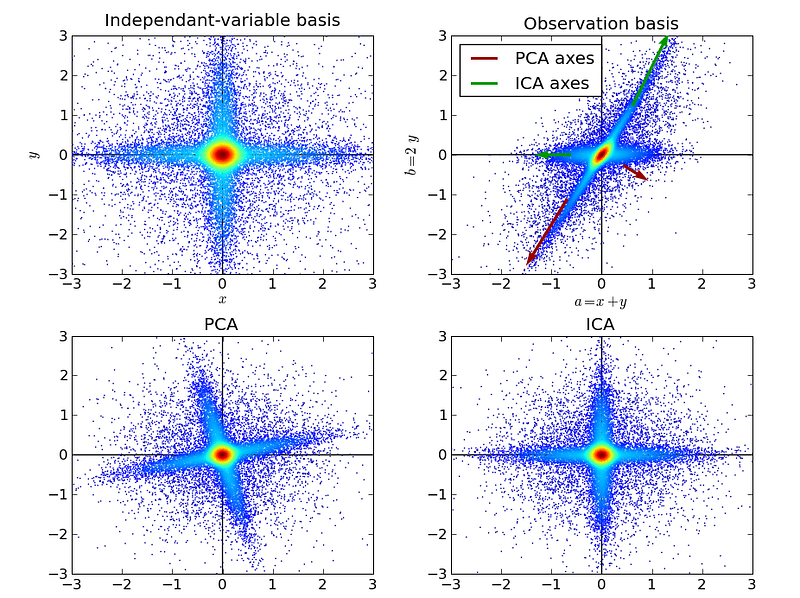Independent Component Analysis
ICA is related to PCA, but it is a much more powerful technique that is capable of finding the underlying factors of sources when these classic methods fail completely. Its applications include digital images, document databases, economic indicators and psychometric measurements.

Now go forth and wield your understanding of algorithms to create machine learning applications that make better experiences for people everywhere.